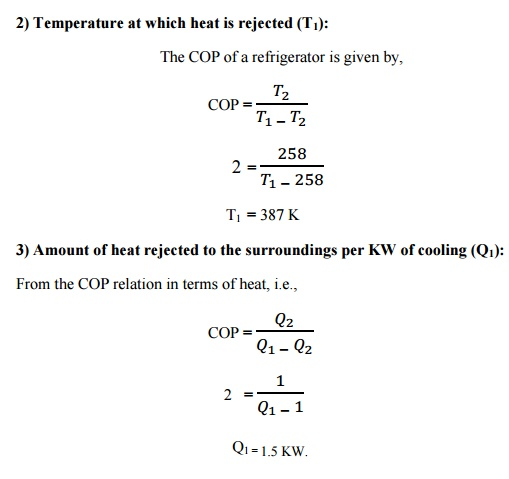Home | | Engineering Thermodynamics | Solved Problems: Thermodynamics Second Law

# Solved Problems: Thermodynamics Second Law

Mechanical - Engineering Thermodynamics - The Second Law of Thermodynamics

1. Two kg of air at 500kPa, 80°C expands adiabatically in a closed system until its volume is doubled and its temperature becomes equal to that of the surroundings which is at 100kPa and 5°C.

For this process, determine

1) the maximum work

2) the change in a availability and

3) the irreversibility.

Take, Cv = 0.718 KJ/kg K, R = 0.287 KJ/kg K.

2. A reversible heat engine receives 3000 KJ of heat from a constant temperature source at 650 K . If the surroundings is at 295 K,

determine

i) the availability of heat energy

ii)  Unavailable heat.A = Q1 –T0 (ΔS)

= 3000 –295 (3.17)

= 2064.85 KJ.

Unavailable heat (U.A) = T0 (ΔS)

= 295 (3.17)

= 935.15 KJ.

Result:

1) The availability of heat energy (A) = 2064.85 KJ.

2) Unavailable heat (U.A) = 935.15 KJ.

3. Air in a closed vessel of fixed volume 0.15 m3 exerts pressure of 12 bar at 250 °C. If the vessel is cooled so that the pressure falls to 3.5 bar , determine the final pressure, heat transfer and change of entropy.

Given Data:

V1 = 0.15 m3

p1 = 12 bar = 1200 KN/m2 p2 = 3.5 bar = 350 KN/m2

T1 = 250°C = 273+250 = 523 K

To find:

1)    The final pressure,

2)    Heat transfer

3)    Change of entropy.

Solution:Result:

1)    The final pressure, T2 = 152.54 K.

2)    Heat transfer, Q = - 446.78 KJ.

3)  Change   of   entropy,-1.06KJ/K.   ΔS   =

4. A domestic food freezer maintains a temperature of - 15°C. The ambient air is at 30°C. If the heat leaks into the freezer at a continuous rate of 1.75 KJ/s, what is the least power necessary to pump the heat out continuously?

Given Data:

TL = - 15°C = 273 –15 = 258 K

TH = 30°C  = 273 + 30 = 303 K

QS = 1.75 KW

To find:

Least power, (W)

Solution:Result:

Least power necessary to pump heat, W = 0.305 KW.

5. A refrigerator working on reversed Carnot cycle requires 0.5 KW per KW of cooling to maintain a temperature of -15°C. Determine the following:

a) COP of the refrigerator

b) Temperature at which heat is rejected and

Amount of heat rejected to the surroundings per KW of cooling.

Given Data:

W = 0.5 KW

Q2 = 1 KW

T2 = -15°C = 273 –15 = 258 K.

To find:

1) COP of the refrigerator (COP)

2) Temperature at which heat is rejected (T1)

3) Amount of heat rejected to the surroundings per KW of cooling (Q1)

Solution:Result:

1) COP of the refrigerator (COP) = 2

2) Temperature at which heat is rejected (T1) = 387 K

Amount of heat rejected to the surroundings per KW of cooling (Q1) = 1.5 KW.

Study Material, Lecturing Notes, Assignment, Reference, Wiki description explanation, brief detail
Mechanical : Engineering Thermodynamics : The Second Law of Thermodynamics : Solved Problems: Thermodynamics Second Law |